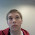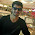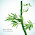### VHDL code for Clock Divider

This is a clock divider code, just set the max-count value as per your requirenment.

For ex. If I want 1Hz freq. set the max count to i/p freq value viz.
1sec = 1Hz
Then, to get time period of 1sec i.e. 1 Hz frequency set max-count to 240000 as shown below:

1sec  =  24000000  -- for i/p frequency of 24 MHz.

To get your desired frequency just calculate the maxcount with the formula given below:

max_count = 24000000 * (1/your required frequency)

CODE:

 ``` 1 2 3 4 5 6 7 8 9 10 11 12 13 14 15 16 17 18 19 20 21 22 23 24 25 26 27 28 29 30 31 32 33 34 35 36 37 38 39 40 41 42 43 44 45 46 47 48``` ```library IEEE; use IEEE.STD_LOGIC_1164.ALL; use IEEE.numeric_std.all; entity clk_div is Port ( Clk : in std_logic; rst : in std_logic; op : out std_logic ); end clk_div; architecture behavioral of clk_div is constant max_count : natural := 24000000; -- I used 24MHz clock begin process(Clk,rst) variable count : natural range 0 to max_count; begin if rst = '0' then count := 0; op <= '1'; elsif rising_edge(Clk) then if count < (max_count/2)-1 then op <='1'; count := count + 1; elsif count < max_count-1 then op <='0'; count := count + 1; else count := 0; op <='1'; end if; end if; end process; end behavioral; ```

 ` `

1.I just wanted to let you know that this has been a huge help. Over the past 4 days, I have been trying to get the on board clock to work by using rippling flip-flops, and I have had no luck. I am new to VHDL, because I have only used it for about 3 weeks, and I want to thank you so very much for taking the time, and showing how to preform the clock divider here on the internet. I would like to ask you for permission to use the code that you have displayed here for my own clock divider, because it will play a valuable role in part of a final project for one of my classes.

2.@Jacob: I am happy that vhdlcodes.com proved helpful to you. Codes are free to use in your projects. If you had any doubts you can share them too. Enjoy programming..!!

3.how would be the modifications if I wish to divide the clk right at the input (in an exsiting module, without making a process or creating a new entity)? Or, is it possible ?

4.If im using 33.333MHz to 1Hz can i use this code??

5.If my board is 33.333MHz can i use this code?? my output must be 1hz also....

6.@Ariya: You will have to use a frequency generator and apply it to input pin for External Clk on your board.

If you want to divide a clock on board theres no other way than using a clk divider 'component' in architecture.

7.@jonykk: Replace the constant value in my code to 33333333. You will get 1Hz o/p.

8.hi jimmy its mak here i need ur help in my uni project could u pls tell me how will i contact u

9.@Malkeet: Hi Malkeet, you can PM me on prasadp4009@gmail.com will give you contact details then.

10.Hi jimmy, i'm looking for a code. were the clock of FPGA is 819khz, N i ve to write a clock divider code n bring it to 1khz.

11.Hello, just set the max_count to 819. And you will get 1KHz..

12.Hi Jimmy, thanks for this. I'm doing my first subject on VHDL and I gotta make a count down timer that when there are two switches to set the time...one makes it go up really quick and one to make it go slower. When there's a button to start the count down. I have tried to incorporate the clock divider her but unfortunately my port mapping skills are naught and just cant get the thing to work. PLS HELP SIR!!! here is my code.....its a bit shoddy but keep in mind that I'm also trying to make it flashes for the last ten seconds....I know I could have used states but unfortunately I dont really know how...............

library ieee;
use ieee.std_logic_1164.all;
use ieee.std_logic_arith.all;
use ieee.std_logic_unsigned.all;

entity counter is

port(clk: in std_logic;
start_SW3:in std_logic;
SW1_fast:in std_logic;
SW2_slow:in std_logic;
LED0:out std_logic_vector (6 downto 0);
LED1:out std_logic_vector (6 downto 0);
LED2: out std_logic_vector (6 downto 0);
LED3: out std_logic_vector (6 downto 0));
attribute loc:string;
attribute loc of clk: signal is "p11";
attribute loc of start_SW3: signal is "p31";
attribute loc of SW1_fast: signal is "p9";
attribute loc of SW2_slow: signal is "p21";
attribute loc of LED0: signal is "p36,p37,p38,p39,p40,p41,p42";
attribute loc of LED1: signal is "p24,p25,p26,p27,p28,p29,p30";
attribute loc of LED2: signal is "p14,p15,p16,p17,p18,p19,p20";
attribute loc of LED3: signal is "p2,p3,p4,p5,p6,p7,p8";

end;

architecture arch_counter of counter is

component SevenSeg
port( LEDin: in integer;
SevenSegOut: out std_logic_vector (6 downto 0));
end component;

signal count0: integer range 0 to 9;
signal count1: integer range 0 to 6;
signal count2: integer range 0 to 11;
signal count3: integer range 0 to 3;

begin

D0: SevenSeg port map(count0, LED0);
D1: SevenSeg port map(count1, LED1);
D2: SevenSeg port map(count2, LED2);
D3: SevenSeg port map(count3, LED3);

13.p_counter: process

begin

if((count3=0) and (count1+count0<11)) then

wait until rising_edge(clk);

if(SW1_fast='1' and SW2_slow='1' and start_SW3='0') then

if((count1=0) and (count0=0)) then
wait until rising_edge(clk);
count1<=5;
count0<=9;
count2<=11;
count3<=count3-1;
elsif(count0=9) then
wait until rising_edge(clk);
count0<=0;
count1<=count1-1;
count2<=11;
elsif((count0=0) and (count1=0) and (count3=0)) then
count1<=0;
count0<=0;
count2<=11;
count3<=0;
else
wait until rising_edge(clk);
count0<=count0-1;
count2<=11;
end if;

elsif(SW1_fast='0' and SW2_slow='1' and start_SW3='1') then

if((count1=0) and (count0=0)) then
wait until rising_edge(clk);
count1<=count1+1;
count0<=count0+1;
count2<=11;
elsif(count0=9) then
wait until rising_edge(clk);
count0<=0;
count1<=count1+1;
count2<=11;
elsif((count0=9) and (count1=5)) then
wait until rising_edge(clk);
count1<=0;
count0<=0;
count2<=11;
count3<=count3+1;
elsif((count0=0) and (count1=0) and (count3=0)) then
count1<=0;
count0<=0;
count2<=11;
count3<=3;
else
wait until rising_edge(clk);
count0<=count0+1;
count2<=11;
end if;
elsif(SW1_fast='1' and SW2_slow='0' and start_SW3='1') then

if((count1=0) and (count0=0)) then
wait until rising_edge(clk);
count1<=count1+1;
count0<=count0+1;
count2<=11;
elsif(count0=9) then
wait until rising_edge(clk);
count0<=0;
count1<=count1+1;
count2<=11;
elsif((count0=9) and (count1=5)) then
wait until rising_edge(clk);
count1<=0;
count0<=0;
count2<=11;
count3<=count3+1;
elsif((count0=0) and (count1=0) and (count3=0)) then
count1<=0;
count0<=0;
count2<=11;
count3<=3;
elsif(SW1_fast='1' and SW2_slow='1' and start_SW3='1') then
count0<=count0;
count1<=count1;
count2<=11;
count3<=count3;
end if;

end if;

else
if(SW1_fast='1' and SW2_slow='1' and start_SW3='0') then

if((count1=0) and (count0=0)) then
wait until rising_edge(clk);
count1<=5;
count0<=9;
count2<=11;
count3<=count3-1;
elsif(count0=9) then
wait until rising_edge(clk);
count0<=0;
count1<=count1-1;
count2<=11;
elsif((count0=0) and (count1=0) and (count3=0)) then
count1<=0;
count0<=0;
count2<=11;
count3<=0;
else
wait until rising_edge(clk);
count0<=count0-1;
count2<=11;
end if;

14.elsif(SW1_fast='0' and SW2_slow='1' and start_SW3='1') then

if((count1=0) and (count0=0)) then
wait until rising_edge(clk);
count1<=count1+1;
count0<=count0+1;
count2<=11;
elsif(count0=9) then
wait until rising_edge(clk);
count0<=0;
count1<=count1+1;
count2<=11;
elsif((count0=9) and (count1=5)) then
wait until rising_edge(clk);
count1<=0;
count0<=0;
count2<=11;
count3<=count3+1;
elsif((count0=0) and (count1=0) and (count3=0)) then
count1<=0;
count0<=0;
count2<=11;
count3<=3;
else
wait until rising_edge(clk);
count0<=count0+1;
count2<=11;
end if;
elsif(SW1_fast='1' and SW2_slow='0' and start_SW3='1') then

if((count1=0) and (count0=0)) then
wait until rising_edge(clk);
count1<=count1+1;
count0<=count0+1;
count2<=11;
elsif(count0=9) then
wait until rising_edge(clk);
count0<=0;
count1<=count1+1;
count2<=11;
elsif((count0=9) and (count1=5)) then
wait until rising_edge(clk);
count1<=0;
count0<=0;
count2<=11;
count3<=count3+1;
elsif((count0=0) and (count1=0) and (count3=0)) then
count1<=0;
count0<=0;
count2<=11;
count3<=3;
elsif(SW1_fast='1' and SW2_slow='1' and start_SW3='1') then
count0<=count0;
count1<=count1;
count2<=11;
count3<=count3;
end if;

end if;
end if;

end process p_counter;

end arch_counter;
library ieee;
use ieee.std_logic_1164.all;
use ieee.std_logic_arith.all;
use ieee.std_logic_unsigned.all;

entity SevenSeg is

port(LEDin: in integer;
SevenSegOut: out std_logic_vector (6 downto 0));

end;

architecture SevenSeg_arch of SevenSeg is

begin
process(LEDin)
begin

Lab0:case LEDin is
when 0=>SevenSegOut<="0000001";
when 1=>SevenSegOut<="1001111";
when 2=>SevenSegOut<="0010010";
when 3=>SevenSegOut<="0000110";
when 4=>SevenSegOut<="1001100";
when 5=>SevenSegOut<="0100100";
when 6=>SevenSegOut<="0100000";
when 7=>SevenSegOut<="0001111";
when 8=>SevenSegOut<="0000000";
when 9=>SevenSegOut<="0000100";
when 11=>SevenSegOut<="1111110";

end case Lab0;

end process;

end SevenSeg_arch;

15.library IEEE;
use IEEE.STD_LOGIC_1164.ALL;
use IEEE.numeric_std.all;

entity sec_clk is
Port (
Clk : in std_logic;
rst: in std_logic;
SW1_fast: in std_logic;
SW2_slow: in std_logic;
start_SW3: in std_logic;
op : out std_logic

);
attribute loc:string;
attribute loc of clk: signal is "p11";
end sec_clk;

architecture RTC of sec_clk is
constant max_count : natural := 2;

component counter

port(op: in std_logic;
start_SW3:in std_logic;
SW1_fast:in std_logic;
SW2_slow:in std_logic;
LED0:out std_logic_vector (6 downto 0);
LED1:out std_logic_vector (6 downto 0);
LED2: out std_logic_vector (6 downto 0);
LED3: out std_logic_vector (6 downto 0));

end component;

signal LED0: std_logic_vector (6 downto 0);
signal LED1: std_logic_vector (6 downto 0);
signal LED2: std_logic_vector (6 downto 0);
signal LED3: std_logic_vector (6 downto 0);

begin
C1: counter port map(op, start_SW3, SW1_fast, SW2_slow, LED0, LED1, LED2, LED3);

compteur : process(Clk,rst)
variable count : natural range 0 to max_count;
begin

if(SW1_fast='1' and SW2_slow='1' and start_SW3='0') then

if rst = '0' then
count := 0;
op <= '1';
elsif rising_edge(Clk) then
if count < max_count/2 then
op <='1';
count := count + 1;
elsif count < max_count then
op <='0';
count := count + 1;
else
count := 0;
op <='1';
end if;
end if;

elsif(SW1_fast='0' and SW2_slow='1' and start_SW3='1') then

if rst = '0' then
count := 0;
op <= '1';
elsif rising_edge(Clk) then
if count < max_count then
op <='1';
count := count + 1;
elsif count < max_count then
op <='0';
count := count + 1;
else
count := 0;
op <='1';

end if;
end if;

elsif(SW1_fast='1' and SW2_slow='0' and start_SW3='1') then

if rst = '0' then
count := 0;
op <= '1';
elsif rising_edge(Clk) then
if count < max_count/4 then
op <='1';
count := count + 1;
elsif count < max_count then
op <='0';
count := count + 1;
else
count := 0;
op <='1';
end if;

end if;
end if;

end process compteur;
end RTC;

1.Hello, Your code seems a bit complicated. You can check my 0 to 9999 updowncode and modify it to countdowntimer.

2.Also use Component declaration instead of declaring all the process in one code. It will make your code more readable and optimized. for dividing clocks you can use the clock divider code as components. Study how components are used in vhdl and you will find the code more easy to write.

3.HI THERE, I WAS WONDERING IF I CAN GET HELP ON HOW TO WRITE A VHDL CODE FOR A TIMER THAT COUNTS DOWN FROM SET TIME OF 4 MINUTS. THE TIME REMAINING IN MINUTES AND SECONDS SHOULD BE SHOWN ON 7 SEGMENT DISPLAY. INPUTS ARE START, STOP, RESET AND OUTPUT MIN AND SECONDS. ALSO A TIME SHOULD BE GIVEN I.E LED FLASHING. THANK YOU FOR YOUR HELP. THIS IS MY FISRT TIME I AM DOING ANYTHING LIKE THIS.

4.Hello Jubel, I am opensourcing the code for count down timer in two days, so keep checking site.

16.HELLO SIR, COULD YOU HELP ME OUT IN WRITING A VHDL CODE FOR A 40 mhz CLOCK TO GENERATE A 1KHZ OUTPUT WITH TON=10 MICROSEC AND TOFF=990 MICROSEC

17.Excellent code. Yes that Vipin guy stole your code.
I tried your code on Nexsys2 Board. My board's clock frequency is 50 MHz.
So I had to use constant max_count : natural := 50000000;
Now, the thing is, I wanted a frequency of 10 MHz so I divided the Max_count by a factor of 5
elsif rising_edge(Clk) then
if count < (max_count/5)-1 then
op <='1';
count := count + 1;
elsif count < (max_count)-1 then
My question is, have I understood the concept properly? Its working on the board. But not sure why I see a wider pulse when I divide by 2 et al.
Sincerely
Sai Kiran Sharma

1.Hello Sai
The max_count/2 actually gives you 50% duty cycle. As you made it /5 so you will get 20% duty cycly signal but with same clock period i.e. you wish to set.

18.Hi! Sir! Could you help me?

19.This comment has been removed by the author.

20.Hi! Sir. I have a problem with my project pump controller with three level water indicator and two motors. Level low and high for motor1 to start and stop. Level medium for motor2. If after motor1 start for 5mn, Water doesn't still reach Level medium, Motor2 starts (there is using water while pumping). Otherwise, motor2 doesn't start. After level high reach, all motors stop.

### Synthesizable RTC in VHDL

The code given is only for clock digit incrementation. For components in clock like min_clk and sec_clk, refer my previous codes.

VHDL code:

### RTC on FPGA with manual set synthesizeble VHDL code.

Hey there, cheers to VHDL. I successfully added manual set feature in my previous RTC project. I am not going to copy whole code here. I am just adding the links of .vhd files. I also added the pre-compiled .sof file only for DE1 boards. There is a very small bug that while clicking the set button to enable min and hr set, it increments the hr or min value unintentionally. Else everything is working great.

(Input freq 24MHz., top-level entity DigiClock)